What does it do?

Checks if any one of the conditions is satisfied or not

Formula breakdown:

=OR(logical1, [logical2], …)

What it means:

Have a couple of conditions that you need to check for and ensure at least one is met? The OR Formula of Excel enforces this and will return TRUE if any one of your required conditions is met!

Let us give this a try in our examples below, we want to check if the date is in the month of January OR the sales amount is greater than \$1000.

I explain how you can do this below:STEP 1: We need to enter the OR function in a blank cell:

## =OR(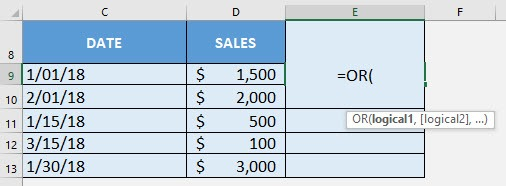STEP 2: The OR arguments:

## logical1

What is the first condition?

Let us create the condition to get the month of the date and check if it is January:

## =OR(MONTH(C9)=1,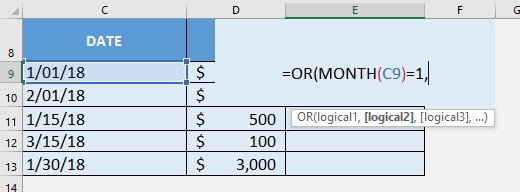## [logical2]

What is the second condition?

Let us create the condition to check if sales is greater than 1000:

## =OR(MONTH(C9)=1, D9 > 1000)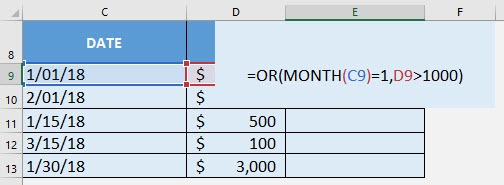Apply the same formula to the rest of the cells by dragging the lower right corner downwards.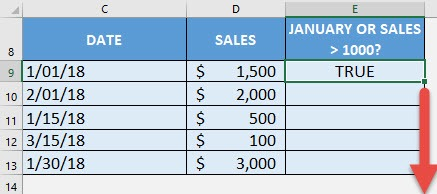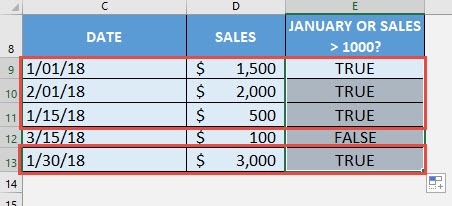How to Use the OR Formula in Excel